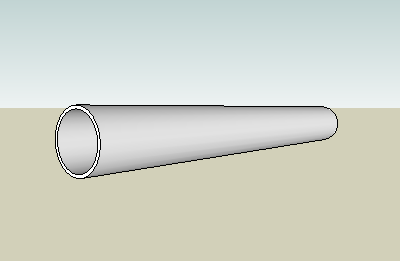Engineering ToolBox - Resources, Tools and Basic Information for Engineering and Design of Technical Applications!

# Circular Ring - Temperature Expansion

## Calculate ring (or pipe) diameter expansion or contraction when temperature changes.The circumference of a thin ring, a pipe or tube, can be expressed as

c0 = 2 π r0                                 (1)

where

c0 = initial circumference (m, inches)

π = 3.14...

r0 = initial radius (m, inches)

The change in circumference due to temperature change can be expressed as

dc = c1 - c

=  2 π r0 dt α                                 (2)

where

dc = change in circumference (m, inches)

c1 = final circumference (m, inches)

dt = temperature change (oC, oF)

α = linear expansion coefficient (mm/moC, μin/inoF)

The final circumference can be expressed as

c1 = 2 π r1                                           (3)

where

r1 = final radius (m, inches)

Equation 1, 2 and 3 can be expressed as

dc = 2 π r1 - 2 π r0

= 2 π r0 dt α

or transformed to

r1 = r0 dt α + r0

= r0 (dt α + 1)                                    (4)

Equation 4 can be modified with diameters to

d1 = d0 (dt α + 1)                                     (5)

### Example - Steel Pipe Diameter Temperature Expansion

A stainless steel pipe with nominal diameter 10 inches (outside diameter 10.750 inches) is heated from 68oF to 98oF. The expansion coefficient for stainless steel S30100 is 9.4 μin/inoF.

The final outside diameter can be calculated

d1 = d0 [dt α + 1]

= (10.750 in) [((98 oF) - (68 oF)) (0.0000094 in/inoF) + 1]

= 10.753 inches

### Circular Ring - Temperature Expansion Calculator

Calculate the final diameter of a thin circular ring after temperature expansion. The calculator can be used for metric and imperial units as long as the use of units are consistent.

d0 - initial diameter (m, mm, in)

dt - temperature difference (oC, oF)

α - temperature expansion coefficient (m/moC, mm/mmoC, in/inoF)

## Related Topics

• Temperature Expansion - Thermal expansion of pipes and tubes - stainless steel, carbon steel, copper, plastics and more.

## Engineering ToolBox - SketchUp Extension - Online 3D modeling!

Add standard and customized parametric components - like flange beams, lumbers, piping, stairs and more - to your Sketchup model with the Engineering ToolBox - SketchUp Extension - enabled for use with the amazing, fun and free SketchUp Make and SketchUp Pro .Add the Engineering ToolBox extension to your SketchUp from the SketchUp Pro Sketchup Extension Warehouse!

Translate

## Privacy

We don't collect information from our users. Only emails and answers are saved in our archive. Cookies are only used in the browser to improve user experience.

Some of our calculators and applications let you save application data to your local computer. These applications will - due to browser restrictions - send data between your browser and our server. We don't save this data.

## Citation

• Engineering ToolBox, (2010). Circular Ring - Temperature Expansion. [online] Available at: https://www.engineeringtoolbox.com/thin-circular-ring-radius-temperature-change-d_1612.html [Accessed Day Mo. Year].

Modify access date.

. .

#### Scientific Online Calculator3 30